Скачать презентацию Large Steam Gas Turbines P M V Subbarao

• Количество слайдов: 26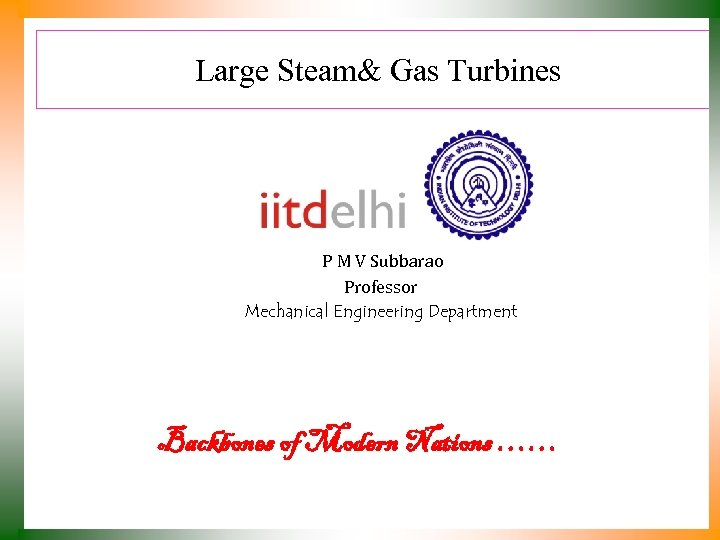Large Steam& Gas Turbines P M V Subbarao Professor Mechanical Engineering Department Backbones of Modern Nations ……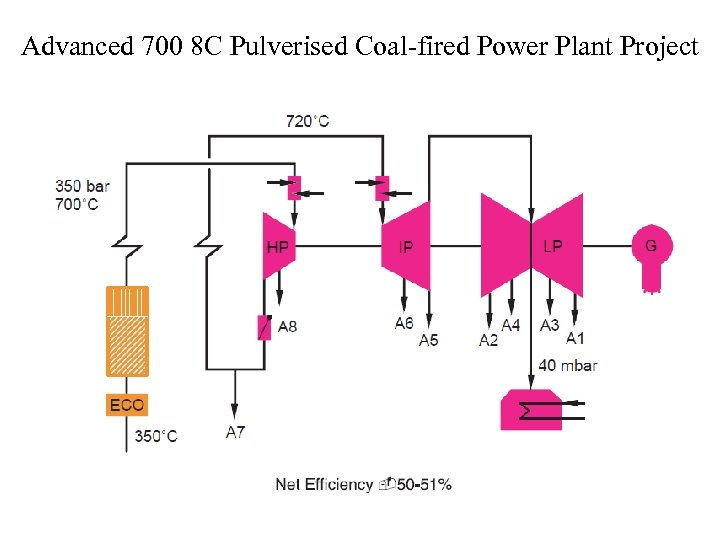Advanced 700 8 C Pulverised Coal-fired Power Plant Project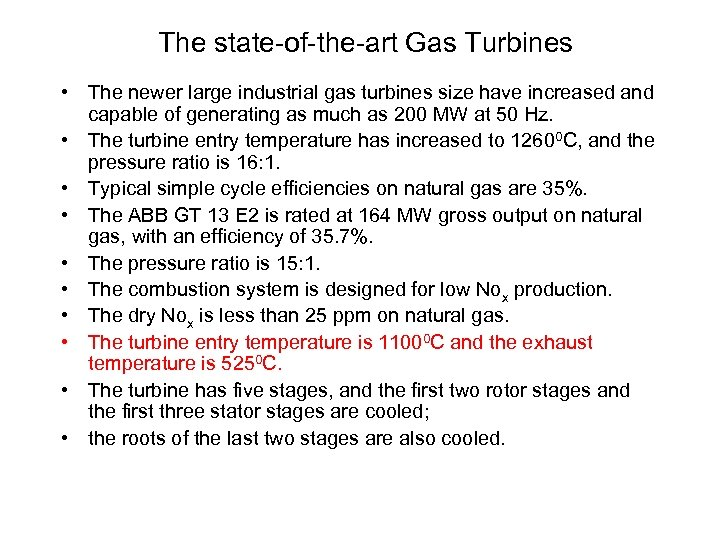The state-of-the-art Gas Turbines • The newer large industrial gas turbines size have increased and capable of generating as much as 200 MW at 50 Hz. • The turbine entry temperature has increased to 12600 C, and the pressure ratio is 16: 1. • Typical simple cycle efficiencies on natural gas are 35%. • The ABB GT 13 E 2 is rated at 164 MW gross output on natural gas, with an efficiency of 35. 7%. • The pressure ratio is 15: 1. • The combustion system is designed for low Nox production. • The dry Nox is less than 25 ppm on natural gas. • The turbine entry temperature is 11000 C and the exhaust temperature is 5250 C. • The turbine has five stages, and the first two rotor stages and the first three stator stages are cooled; • the roots of the last two stages are also cooled.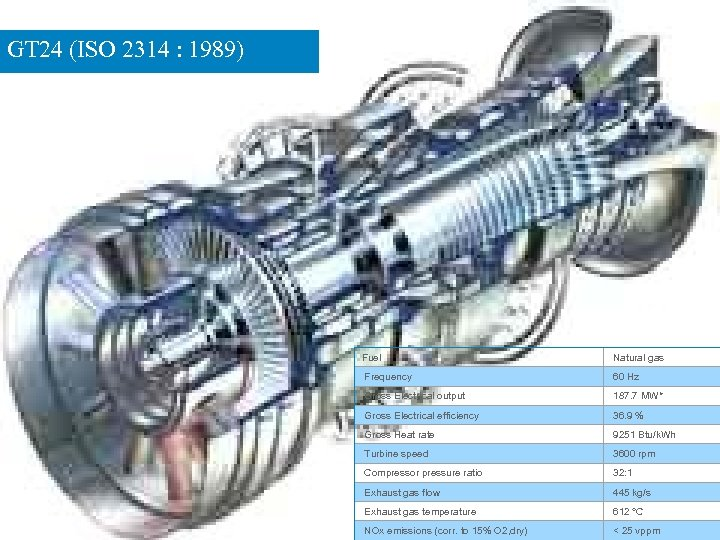GT 24 (ISO 2314 : 1989) Fuel Natural gas Frequency 60 Hz Gross Electrical output 187. 7 MW* Gross Electrical efficiency 36. 9 % Gross Heat rate 9251 Btu/k. Wh Turbine speed 3600 rpm Compressor pressure ratio 32: 1 Exhaust gas flow 445 kg/s Exhaust gas temperature 612 °C NOx emissions (corr. to 15% O 2, dry) < 25 vppm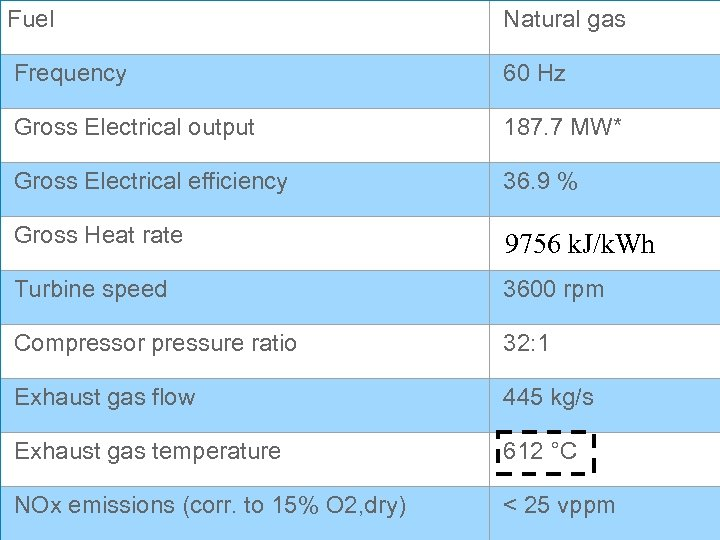Fuel Natural gas Frequency 60 Hz Gross Electrical output 187. 7 MW* Gross Electrical efficiency 36. 9 % Gross Heat rate 9251 Btu/k. Wh 9756 k. J/k. Wh Turbine speed 3600 rpm Compressor pressure ratio 32: 1 Exhaust gas flow 445 kg/s Exhaust gas temperature 612 °C NOx emissions (corr. to 15% O 2, dry) < 25 vppm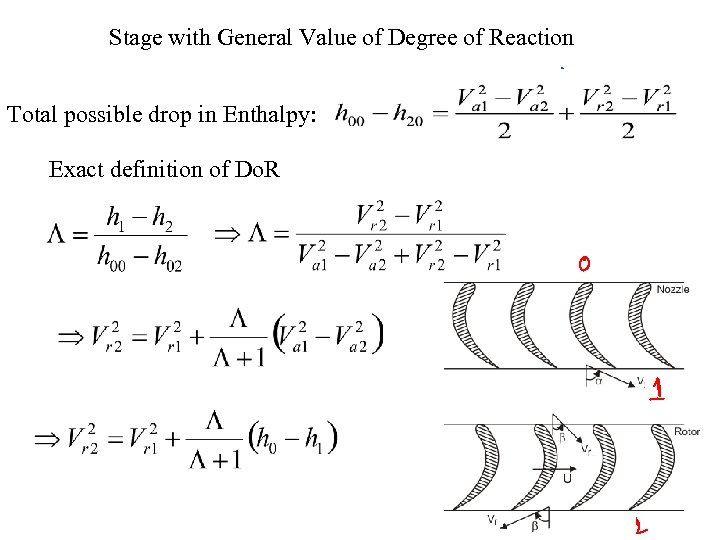Stage with General Value of Degree of Reaction Total possible drop in Enthalpy: Exact definition of Do. R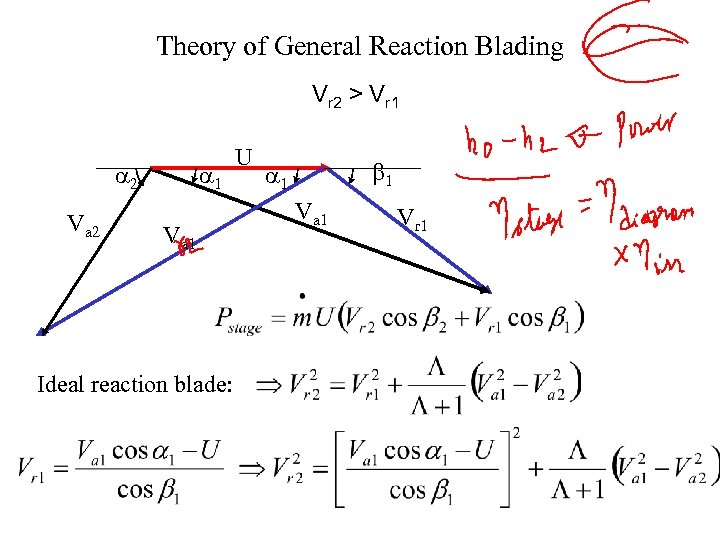Theory of General Reaction Blading Vr 2 > Vr 1 a 2 Va 2 a 1 Va 1 Ideal reaction blade: U b 1 a 1 Vr 1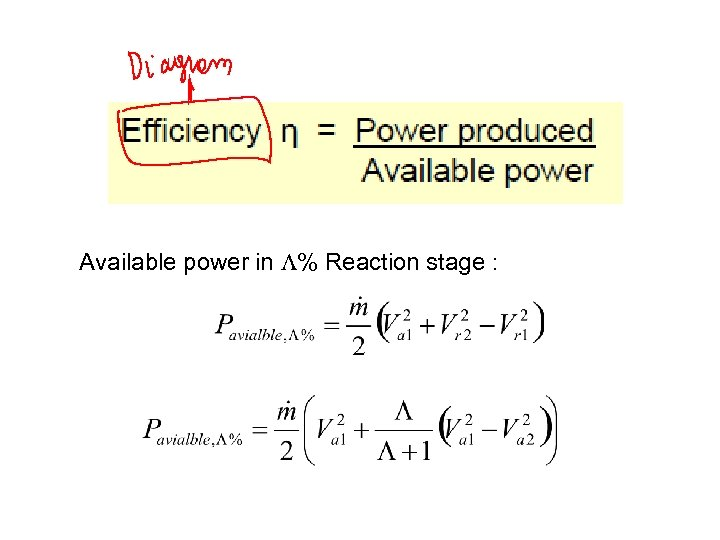Available power in L% Reaction stage :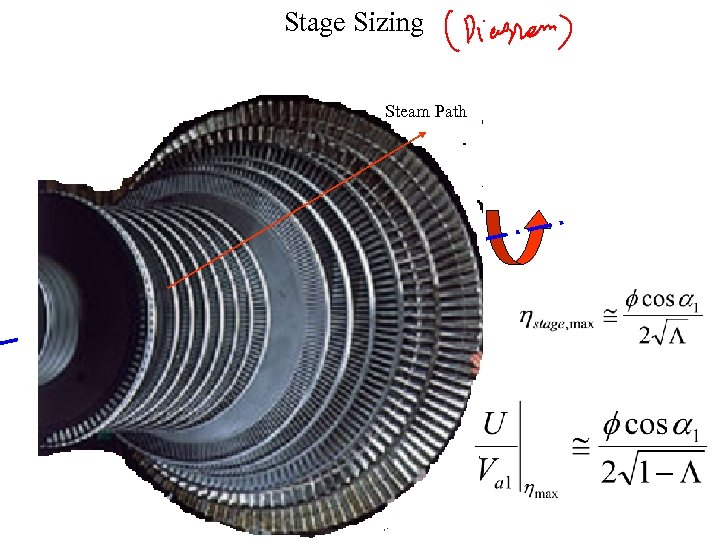Stage Sizing Steam Path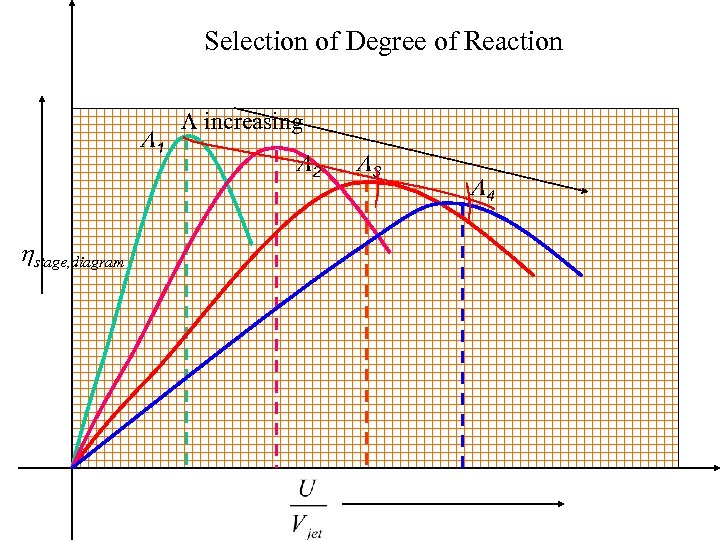Selection of Degree of Reaction L 1 hstage, diagram L increasing L 2 L 3 L 4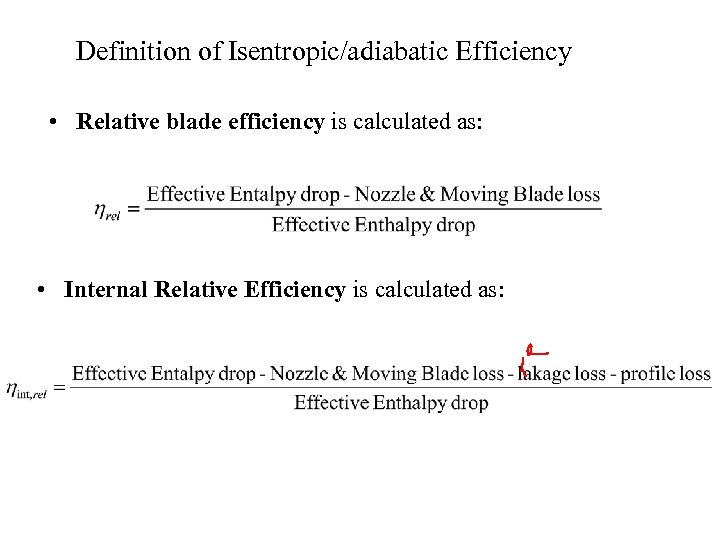Definition of Isentropic/adiabatic Efficiency • Relative blade efficiency is calculated as: • Internal Relative Efficiency is calculated as: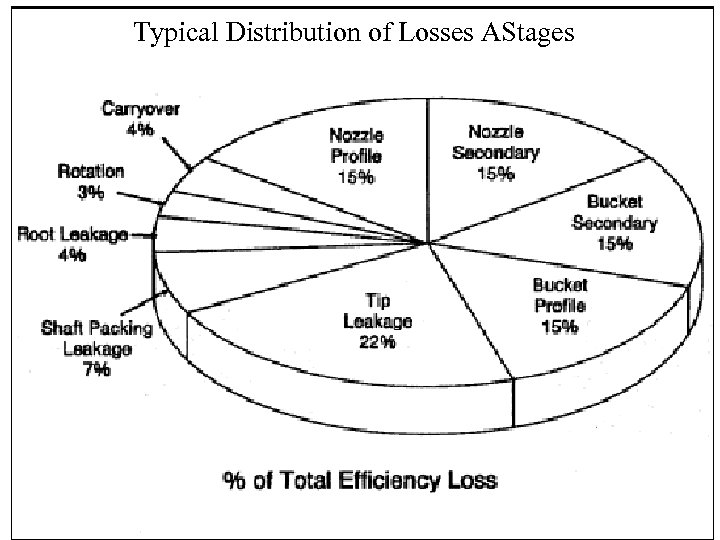Typical Distribution of Losses AStages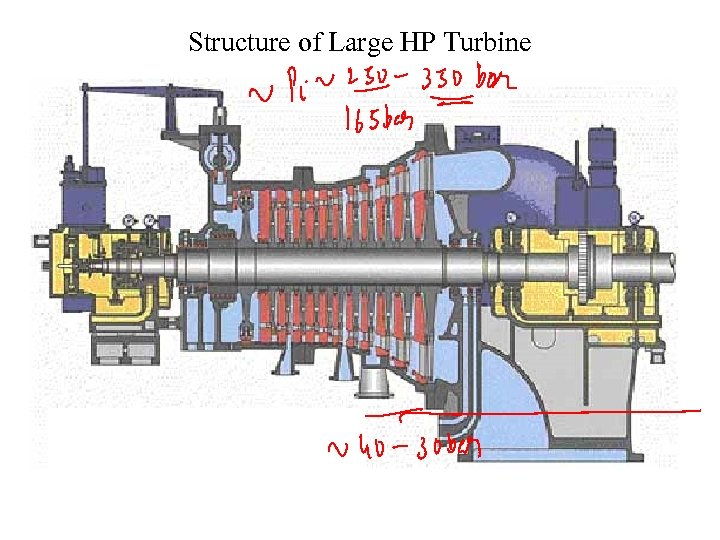Structure of Large HP Turbine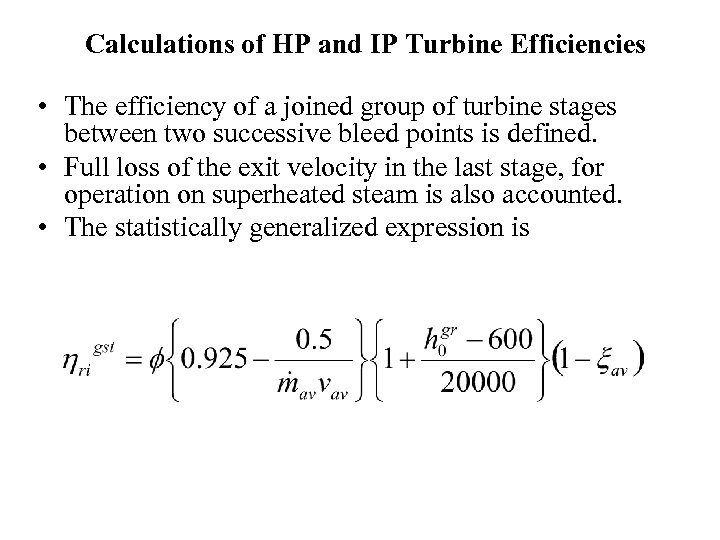Calculations of HP and IP Turbine Efficiencies • The efficiency of a joined group of turbine stages between two successive bleed points is defined. • Full loss of the exit velocity in the last stage, for operation on superheated steam is also accounted. • The statistically generalized expression is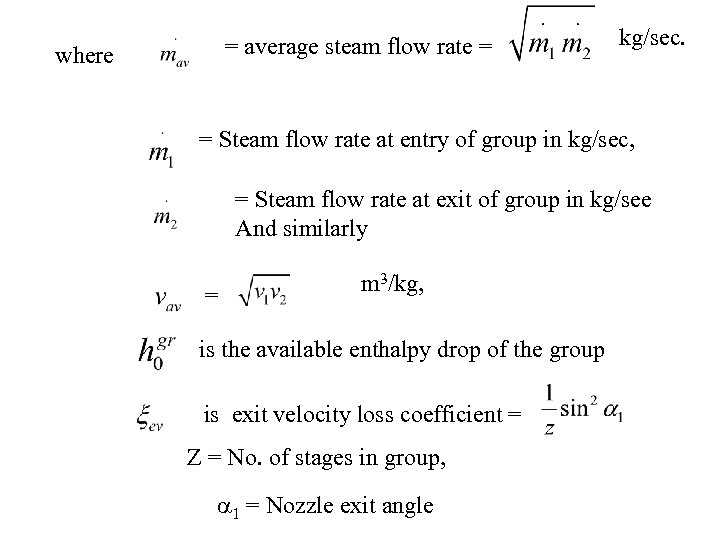= average steam flow rate = where kg/sec. = Steam flow rate at entry of group in kg/sec, = Steam flow rate at exit of group in kg/see And similarly = m 3/kg, is the available enthalpy drop of the group is exit velocity loss coefficient = Z = No. of stages in group, a 1 = Nozzle exit angle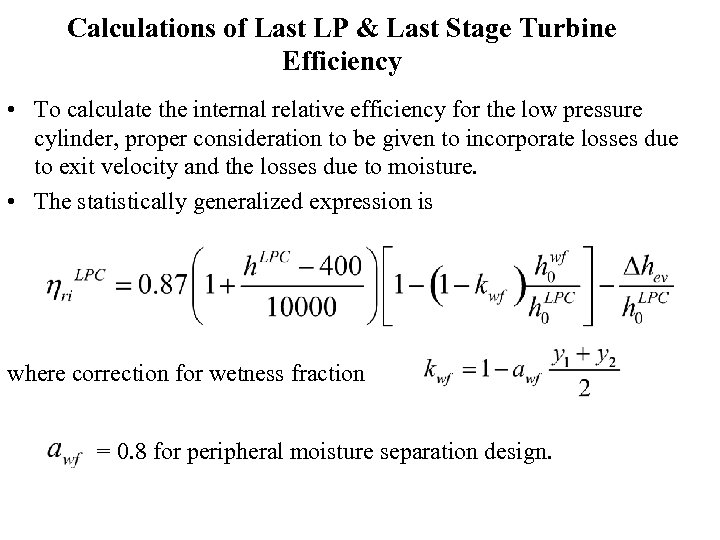Calculations of Last LP & Last Stage Turbine Efficiency • To calculate the internal relative efficiency for the low pressure cylinder, proper consideration to be given to incorporate losses due to exit velocity and the losses due to moisture. • The statistically generalized expression is where correction for wetness fraction = 0. 8 for peripheral moisture separation design.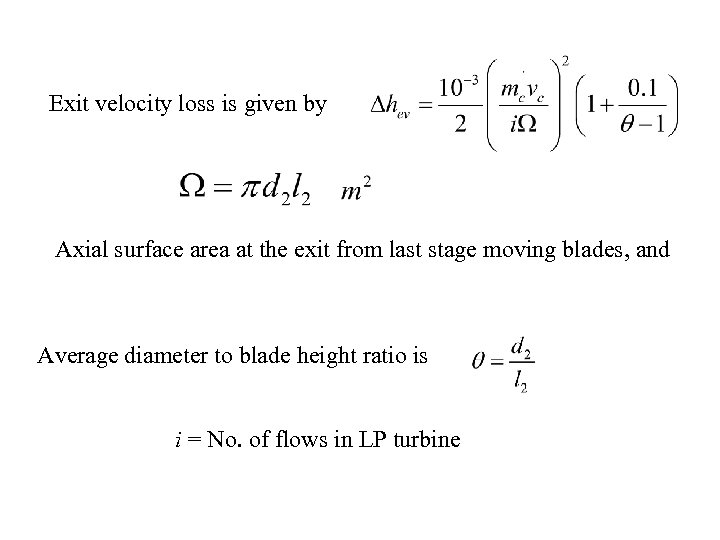Exit velocity loss is given by Axial surface area at the exit from last stage moving blades, and Average diameter to blade height ratio is i = No. of flows in LP turbine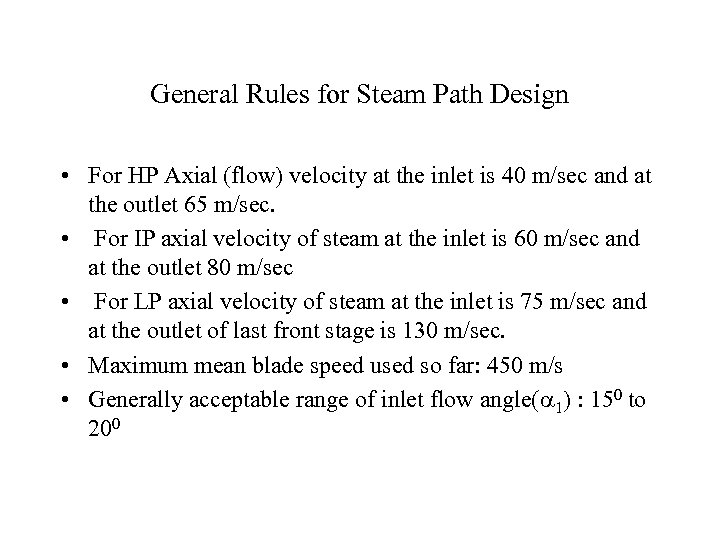General Rules for Steam Path Design • For HP Axial (flow) velocity at the inlet is 40 m/sec and at the outlet 65 m/sec. • For IP axial velocity of steam at the inlet is 60 m/sec and at the outlet 80 m/sec • For LP axial velocity of steam at the inlet is 75 m/sec and at the outlet of last front stage is 130 m/sec. • Maximum mean blade speed used so far: 450 m/s • Generally acceptable range of inlet flow angle(a 1) : 150 to 200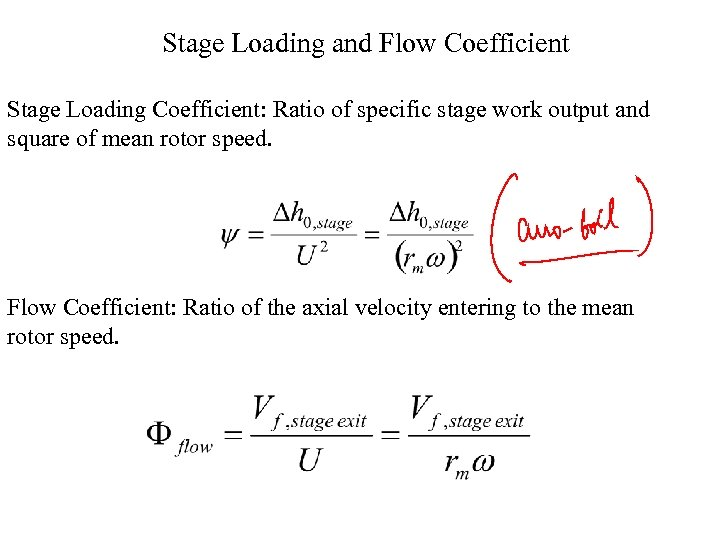Stage Loading and Flow Coefficient Stage Loading Coefficient: Ratio of specific stage work output and square of mean rotor speed. Flow Coefficient: Ratio of the axial velocity entering to the mean rotor speed.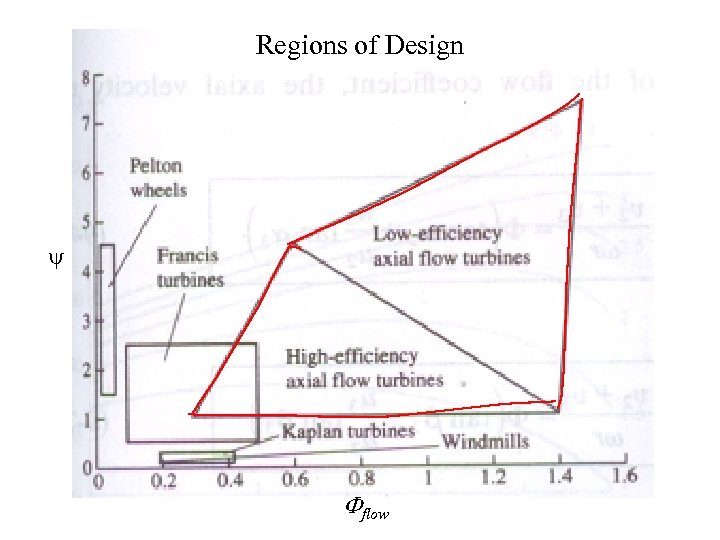Regions of Design y Fflow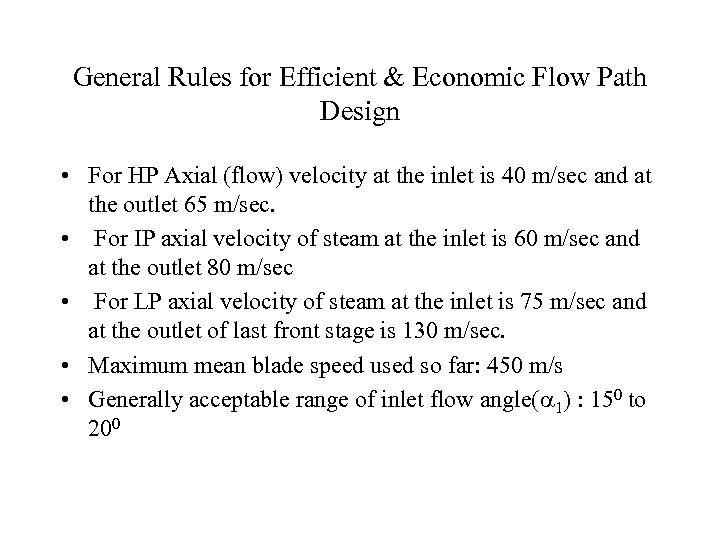General Rules for Efficient & Economic Flow Path Design • For HP Axial (flow) velocity at the inlet is 40 m/sec and at the outlet 65 m/sec. • For IP axial velocity of steam at the inlet is 60 m/sec and at the outlet 80 m/sec • For LP axial velocity of steam at the inlet is 75 m/sec and at the outlet of last front stage is 130 m/sec. • Maximum mean blade speed used so far: 450 m/s • Generally acceptable range of inlet flow angle(a 1) : 150 to 200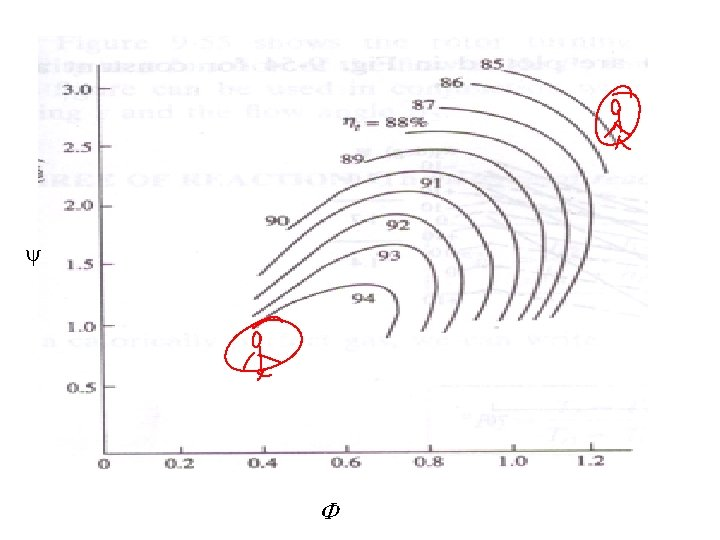y F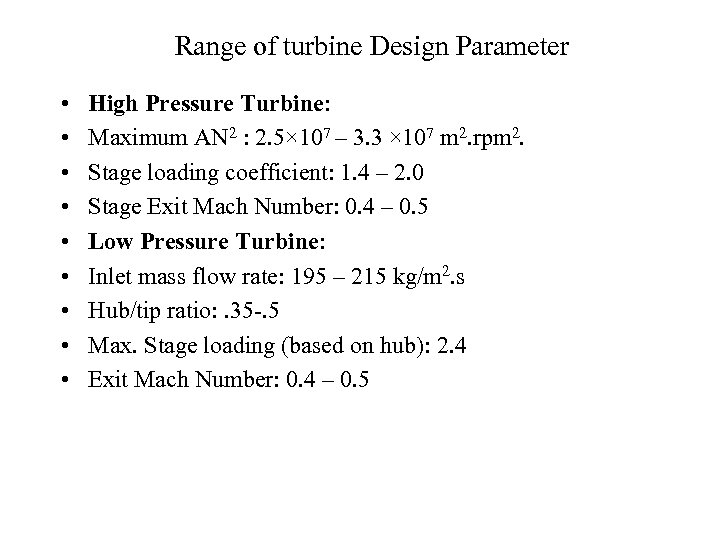Range of turbine Design Parameter • • • High Pressure Turbine: Maximum AN 2 : 2. 5× 107 – 3. 3 × 107 m 2. rpm 2. Stage loading coefficient: 1. 4 – 2. 0 Stage Exit Mach Number: 0. 4 – 0. 5 Low Pressure Turbine: Inlet mass flow rate: 195 – 215 kg/m 2. s Hub/tip ratio: . 35 -. 5 Max. Stage loading (based on hub): 2. 4 Exit Mach Number: 0. 4 – 0. 5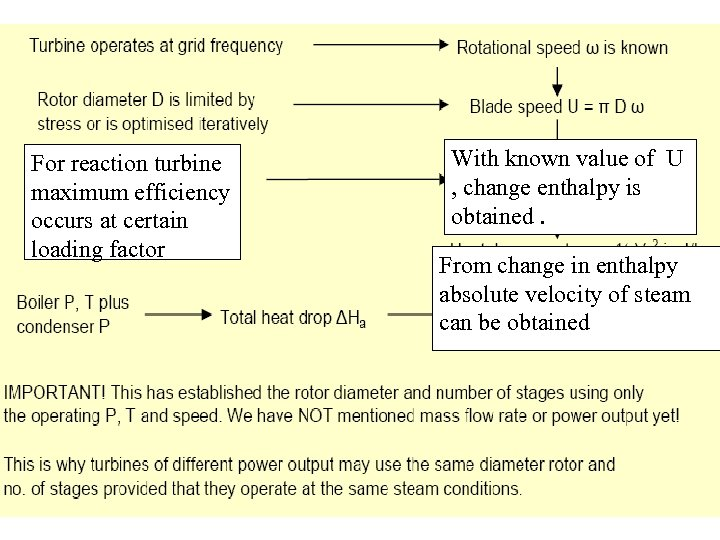For reaction turbine maximum efficiency occurs at certain loading factor With known value of U , change enthalpy is obtained. From change in enthalpy absolute velocity of steam can be obtained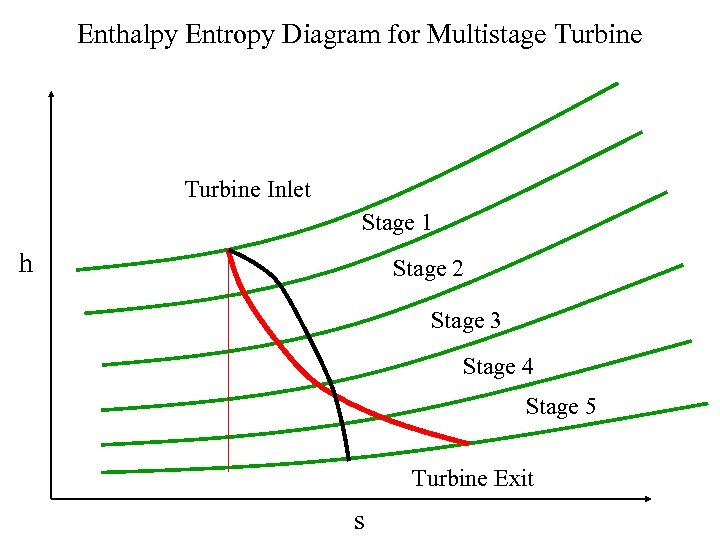Enthalpy Entropy Diagram for Multistage Turbine Inlet Stage 1 h Stage 2 Stage 3 Stage 4 Stage 5 Turbine Exit s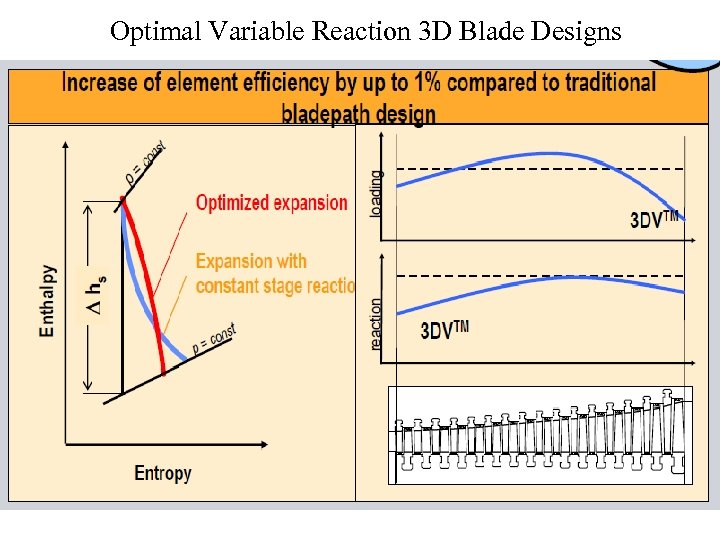Optimal Variable Reaction 3 D Blade Designs